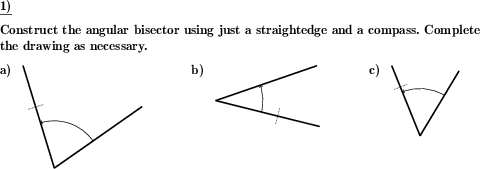Custom math worksheets at your fingertips# Details for problem "Angle bisector construction"

Quickname: 9432

Elementary School, Primary School, Junior High School, Middle School, High School.

## Summary

Construct the bisector for a given angle using just a compass and a straightedge.

## Example## Description

A drawing of an angle is given. The task is to do construct its bisector in with the classic straightedge and compass construction, also known as ruler-and-compass construction. The construction follows these steps:

1. Two arcs are made on each ray of the angle with the same compass opening, which means the two points that are marked on the two rays of the angle have the same distance to the angle's vertex.
2. From each of the marked points on the angle's rays swing an arc between the rays, making sure these arcs intersect at some point.
3. Draw a line from the angle's vertex through the newly formed arc intersection. This line is the angle's bisector.

For an easier problem statement, in addition to the angle itself, other construction elements may be provided:

• a single arc on one of the angle's rays
• both arcs on the angle's rays
• two arcs, one on one of the rays and the corresponding arc that has been swung originating from the respective point on the ray
• all of the arcs that have to be swung, so all left to do is to draw the bisector itself

The angle's size can be chosen to be up to 90 degrees or up to 170 degrees. The size of the drawing can selected from multiple options. There will always be enough space left on the sheet to allow the full construction.

Download free printable worksheets for this math problem here. The worksheet contains the problems only, the solution sheet includes the answers. Just click on the respective link.

•Worksheet 1Solution sheet with answers
•Worksheet 2Solution sheet with answers
•Worksheet 3Solution sheet with answers

If you can not see the solution sheets for download, they may be filtered out by an ad blocker that you may have installed. If this is the case, please allow ads for this page and reload the page. The solution sheets will then reappear.

• Do these sample worksheets do not really fit?
• Do you need more math worksheets, with a different level of difficulty?
• Would you like to combine different problems on a worksheet and adjust them to your needs?
• As a teacher, you can put together your own worksheets using the automatically generated math problems provided.
With a free initial credit, you can start creating your own math worksheets in a few minutes.

You can try it for free! Register here, to create custom worksheets now!

## Customization options for this problem

Parameter
Possible values
Number of problems
1, 2, 3, 4, 5
Size of drawing
4cm, 6cm, 8cm, 10cm, 12cm
Given elements
none, one arc on single ray, arcs on both rays, both arcs, one side, all arcs
Max size of angle
90, 170

## Similar problems

Remark
Description
Angle bisectors in a triangle
Draw heights, medians, angle bisectors or perpendicular bisectors for a triangle.